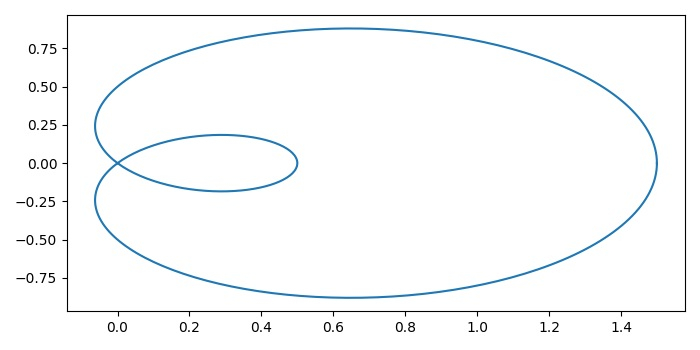# Draw parametrized curve using pyplot.plot() in Matplotlib

To draw a parametrized curve using pyplot.plot(), we can take the following Steps −

• Set the figure size and adjust the padding between and around the subplots.

• Initialize a variable N for the number of samples.

• Create t, r, x and y data points using numpy.

• Create a figure and a set of subplots.

• Use plot() method to plot x and y data points.

• To display the figure, use show() method.

## Example

import numpy as np
import matplotlib.pyplot as plt
plt.rcParams["figure.figsize"] = [7.00, 3.50]
plt.rcParams["figure.autolayout"] = True

N = 400
t = np.linspace(0, 2 * np.pi, N)
r = 0.5 + np.cos(t)
x, y = r * np.cos(t), r * np.sin(t)
fig, ax = plt.subplots()
ax.plot(x, y)
plt.show()

## Output